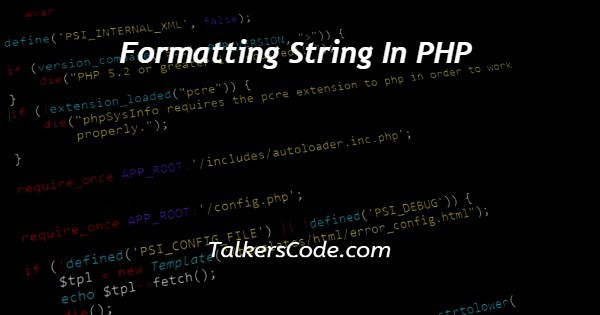# Formatting String In PHP

Last Updated : Jan 1, 2023In this tutorial we will show you the solution of formatting string in PHP, formatting as we know is a part of type conversion and for formatting we need formatting specifiers.

In php we had more type of formatting specifiers like ‘%f or %x’.

Here we used all specifiers with example so user can easily understand which specifier how will work where we can use them.

For format and print those converted string we can use sprint() or printf() any one method.

## Step By Step Guide On Formatting String In PHP :-

Here we defined variable \$num and stored value ‘100’ on that then we printing original number before formatting on screen using echo() method.

We printed heading for formatting string result then we converted value ‘100’ to binary, ascii, float, octal and hexadecimal formatted types using printf() method.

Those each formatting done by symbols ‘%b,%c,%f,%o,%x’ when one variable referred by these symbols original value converted to respective formats.

```<?php
\$num=100;
echo("Original Number: ".\$num."<br>");
echo "Formatted Strings<br>";
printf("Binary: %b<br>",\$num);
printf("ASCII: %c<br>",\$num);
printf("Float: %f<br>",\$num);
printf("Octal: %o<br>",\$num);
?>```
1. A php script can be placed anywhere in the document. A php script starts with <?php and end with ?>.
2. The default file extension for php files is “.php” and php statements end with ‘;’ semicolon.
3. We defined variable \$num and stored value ‘100’ on it. Using echo() method we printing variable ‘\$num’ value before formatting then we printed another message for instruct users.
4. Then we used printf() method for conversion of string formats. First we converting variable \$num into binary format by symbol %b and also we can easily know binary value for decimal 100 value.
5. Next we converting variable \$num into ASCII format by symbol %c and also we can easily know ASCII value for decimal 100 value.
6. Then we converting variable \$num into Float format by symbol %f and also we can easily know float value for decimal 100 value.
7. Next we converting variable \$num into octal format by symbol %x and also we can easily know octal value for decimal 100 value.
8. We converting variable \$num into hexadecimal format by symbol %x and also we can easily know hexadecimal value for decimal 100 value.
9. In printf() method %b refers binary format and we need binary format for number ‘100’ so we used ‘\$num’ variable as parameter going to convert as binary likewise each one formats done.

## Conclusion :-

In conclusion we are able to know how to format string in php. First we need to start our xampp server then we load this program on browser we can see result of original and formatted string conversions.

In future we need to use anyone format of string so we have to know about them.

That symbol ‘%’ next each character refers each formats. We can also use same concept for different decimal value we can get result for the respected defined number.

I hope this tutorial on formatting string in PHP helps you and the steps and method mentioned above are easy to follow and implement.